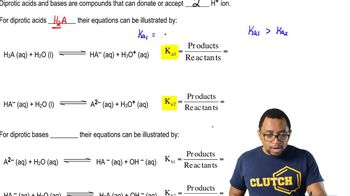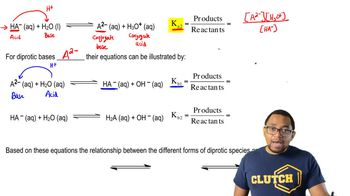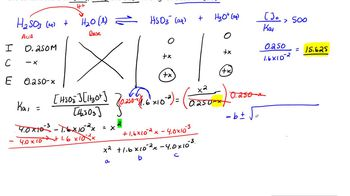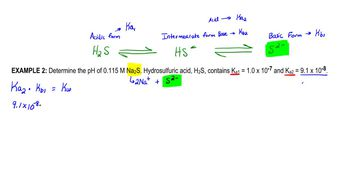Start typing, then use the up and down arrows to select an option from the list.# Diprotic Acids

Jules Bruno
212views
1
So based on what we've seen so far, we're gonna say, based on these equations, the relationship between the different forms off died, product species are all right. So before it's given away any of its H plus values, it's going to be Htwe. So you could think of this as it's acidic form. When he gives away the first H plus, it has become H A minus. We'll call this the intermediate form and then we have it giving away its second H plus to become a to minus. So it's lost. All of its H plus is now, so this would be its basic form. These would be the three major forms of any dye product acid or base. Now, as a result of having multiple K s and KBS, we can't just simply say anymore that K Times K B equals K w. Now you have to remember that K one is connected to K B two and multiplying them together gives us our K W, which is still 1.0 times 10 to the negative 14 when the temperature is 25 degrees Celsius and then remember that a k A to is connected to k B one and they're both still connected to K. W. Now, why is that? Well, if we take a look here at the three forms, we're gonna say we're going from the full it fully acid acid form. And we're giving away our first H plus to give us our intermediate form. So the relationship between them is connected by K one. Then if we're giving away the second acidic hydrogen, that's gonna give us K two, and that's what we're looking at it this way. Now, if we look at it the opposite way going this way, this is its basic form in the very beginning, and it accepts in H Plus to become this form. If we're talking about it accepting its first H plus, we're dealing with CBI one. So notice here that K B one and K two are connected here. Then if we're talking about accepting our second H plus to give us back our acidic form, that means we're talking about CBI, too. So that's why K one and K B two are connected, and that's why K two and K B one are connected. So just remember the relationships between those acid Constance and based Constance. Now we're gonna stay here when dealing with di product acids. What? We're trying to figure out our pH. We're gonna say H two way can be treated as the mono product acid in the same way and in that case would use K one. Then, for the intermediate form, we would use K two because it give away its second acidic hydrogen to become just like the base. Then we'd say that a to minus represents the basic foreman in that case would use K B one and technically, for the intermediate form, we could use either que two or k B two. Depending on. Are we is this thing accepting an H plus? If it's accepting an H plus, then we'd use K B two. Is it giving away an H plus? In that case, we use K two, so the intermediate forms a little bit tricky. It's all based on. Is it acting as an acid, or is it acting as a base? Because, remember, here it has ah, hydrogen in front and a negative charge in the back so it can act as either an acid or base because it is anfo Terek. And because of that, we could go and use K two if it's acting, hacking acid and donating an H plus. Or we could use K B two if it's gonna accept an H plus and act like a base. So these are just some of the fundamental principles you need to keep in mind when dealing with di product acids and bases. As we go further into this concept will look at problems and we'll learn. Learn how to approach those different types of questions to figure out what our pH or P O. H will be.03:4302:2304:1413:3406:41